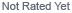Loading... Please wait...

# Choosing The Correct Solar Panel

## Choosing The Correct Solar PanelThis is the fourth and final article in a series that we’ve written as a guide to choosing a solar panel for a stand-alone solar energy system with battery storage. If you’ve been reading along, you know that the basic process of choosing the solar panel involves 3 steps:

Step 1. Calculate what your load needs to run for a day. Step 2. Find out how much sun you’ll get in a day at your location.
Step 3. Calculate which solar panel will run the load with that amount of sun.

We’ve done a lot of explaining to get to Step 3, because it can get confusing for a first-timer to figure out which numbers to use in the calculations in Step 1 and Step 3. Solar panels are rated using a value called Wmp (the maximum power produced by the panel), but there are some problems with using the Wmp rating of a solar panel in the calculations. We talked about these problems in the earlier articles in this series.

One problem (that you can’t really do anything about) is that the test conditions that the power rating Wmp is based on are out-of-the box lab conditions, and they may be 10-15% higher than what a panel will produce in the real world over its lifetime.

A second problem is that the power rating Wmp is actually calculated from two other ratings, the voltage rating and the current rating of the panel. The problem here arises because the voltage that a solar panel produces at max power (Vmp) is higher than the voltage at which a battery actually charges. Vmp is usually about 17.5V, but a 12V battery charges at about 14V. And, the device that you want to run off the battery runs at about 12V. These differences in voltages cause a problem with the calculations.

But there is something you can do to get around this. What you have to do, basically, is leave the voltage out of the calculations in Steps 1-3. You can do this by using the current rating (Imp) of the solar panel to calculate something called an Ah. An Ah is a unit used to measure the capacity, or state of charge, of the battery. To calculate how much charge a solar panel can put into a battery in a day, use the revised steps below:

Revised Step 1. Calculate the Ah per day needed by the load
Revised Step 2. Choose a Peak Sun Hour value from our solar insolation maps, in hours per day
Revised Step 3. Calculate which solar panel will provide the Ah per day that you need

Now here’s an example of how to do this: Let’s say you want to run a 12V, 10W bulb for 5 hours/day:

Step 1: 10W bulb X 5 hours/day = 50 watt-hours/day – that’s how much energy your bulb will use in a day. The bulb will be running at 12V, so divide 50 Wh by 12V. 50/12 = 4.17, so the bulb will use 4.17Ah in a day.

Step 2: Look at a solar insolation map to find the number of Peak Sun Hours at your location, and at the time of year that you want to run the lightbulb. If you want to run the lightbulb all through the year, use the Peak Sun Hour value for the lowest winter month. For example, in New Hampshire there are about 2.5 Peak Sun Hours per day in December. Use this number in Step 3.

Step 3: Now divide the Ah that you need (from Step 1) by the number of Peak Sun Hours per day: 4.17Ah/2.5h = 1.7A

This means that a panel that produces 1.7A is what you want. A 30W solar panel produces 17.5V at about 1.7A. That’s the panel to buy!

Now let’s go back and see what you would have calculated using the original 3 steps with the Wmp solar panel rating. Step 1: The load uses the same 50 Wh per day. Step 2: Use the same 2.5 Peak Sun Hours Step 3: 50Wh/2.5 Peak Sun Hours = 20W.

These calculations would have told you that you need only a 20W panel, when in fact you need a 30W panel! Actually, adding the 10-15% that you need to take into account the real world conditions, you’d want more like a 35W panel.

In the next newsletter, we’ll talk about sizing a battery bank to create a reliable system that will always work when you need it.

## New Products

•••••